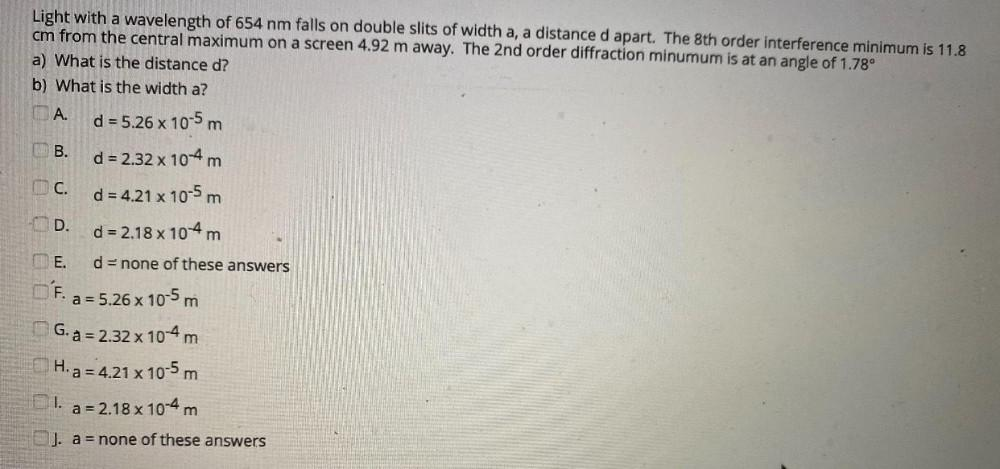Question:

# -D Light with a wavelength of 654 nm falls on double slits of width a, a distance d apart. The 8th order interference minimum is-D Light with a wavelength of 654 nm falls on double slits of width a, a distance d apart. The 8th order interference minimum is 11.8 cm from the central maximum on a screen 4.92 m away. The 2nd order diffraction minumum is at an angle of 1.78° a) What is the distance d? b) What is the width a? A. d = 5.26 x 10-5 m B. d = 2.32 x 10-4 m d = 4.21 x 10-5 m d = 2.18 x 104 m THE. d = none of these answers O'F. a = 5.26 x 10-5 m G. a = 2.32 x 10-4 m H. a = 4.21 x 10-5 m 1. a = 2.18 x 10-4 m J. a = none of these answers